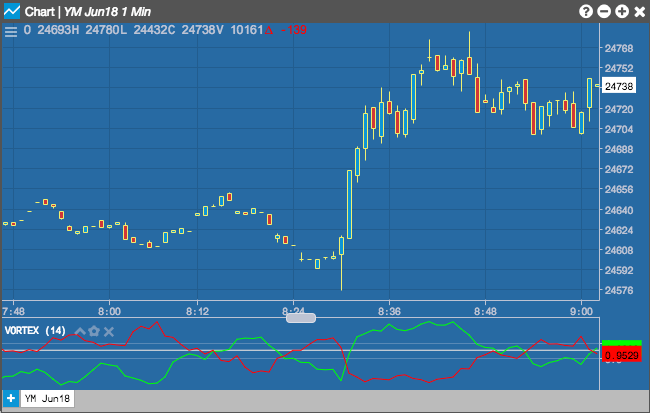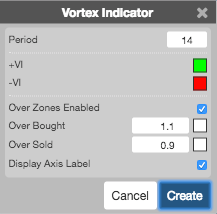Charts

# Vortex Indicator

The Vortex Indicator consists of two oscillators (VI+ and VI-) used for identifying the start and direction of a market trend. When the VI+ and VI- cross, it generally indicates a market trend. The trend is up when VI+ stays above VI-, and the market trend is down when VI- crosses below VI+.## Configuration Options• Period: Number of bars to use in the calculations.
• Color Selectors: Colors to use for graph elements.
• Over Zones Enabled: Whether to shade the area between the plot and the horizontal overbought and oversold levels.
• Over Bought: Overbought quantity
• Over Sold: Oversold quantity
• Display Axis Label: Whether to display the most recent value on the Y axis.

## Formula

The calculation for the indicator is divided into three parts:

1. Uptrend and downtrend movement:
• $$VM+ = {Current High} - {Prior Low}$$
• $$VM- = CurrentLow - PriorHigh$$
• $$\sum_{n=1}^{x} VM\verb "+"$$
• $$\sum_{n=1}^{x} {VM\verb "-"}$$
Where "x" is the number of price bars or periods the indicator will look at (between 14 and 30 is common).
2. True Range (TR) is the greatest of:
• $$CurrentHigh - CurrentLow$$
• $$\left | CurrentHigh - PrevioiusClose \right |$$
• $$\left | CurrentHigh - CurrentLow \right |$$
• $$\left | CurrentHigh - CurrentLow \right |$$
3. Normalize uptrend downtrend movement:
• $$VIx+ = \frac{VMx+}{TRx}$$
• $$VIx- = \frac{VMx-}{TRx}$$